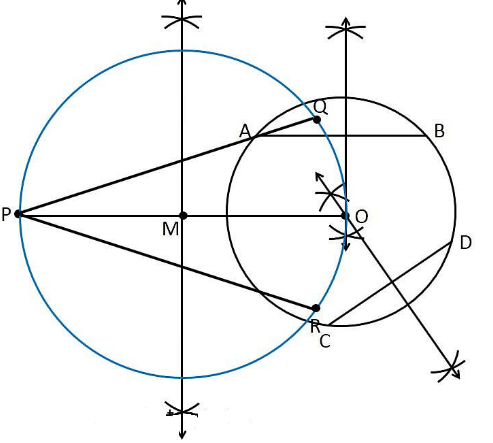# Draw a circle with the help of a bangle. Take a point outside the circle. Construct the pair of tangents from this point to the circle. Give the justification of the construction

To construct a tangent from given conditions

1. Let us draw a circle with the help of a bangle.

2. Now draw two non-parallel chords such as AB and CD

3. Draw the perpendicular bisector of AB and CD

4. Consider the centre as O where the perpendicular bisector intersects.

5. To draw the tangents, take a point P outside the circle.

6. Join the points O and P.

7. Now draw the perpendicular bisector of the line PO and midpoint is taken as M

8. Considering M as centre and MO as radius draw a circle.

9. Let the circle intersects intersect the circle at the points Q and R

10. Now join PQ and PR

11. Therefore, PQ and PR are the required tangents.Justification

We have to prove that PQ and PR are the tangents to the circle.

Since, O is the centre of a circle, we know that the perpendicular bisector of the chords passes through the centre.

Now, join the points OQ and OR.

We know that the perpendicular bisector of a chord passes through the centre.

It is clear that the intersection point of these perpendicular bisectors is the centre of the circle.

Since ∠PQO is an angle in the semi-circle. We know that an angle in a semi-circle is a right angle.

∴ ∠PQO = 90°

⇒ OQ⊥ PQ

Since OQ is the radius of the circle, PQ has to be a tangent of the circle. Similarly,

∴ ∠PRO = 90°

⇒ OR ⊥ PO

Since OR is the radius of the circle, PR has to be a tangent of the circle

Therefore, PQ and PR are the required tangents of a circle.